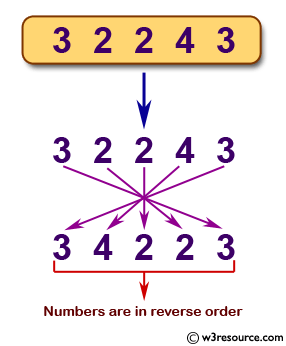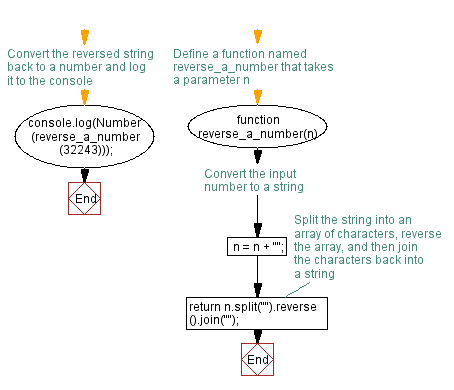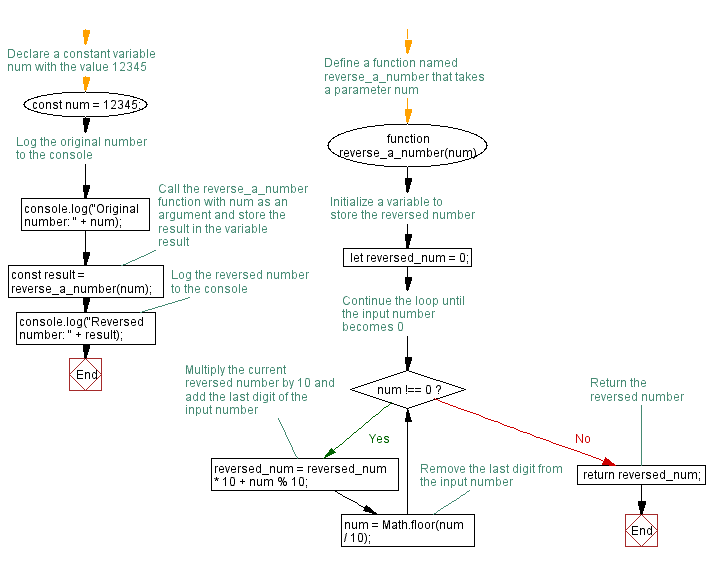# JavaScript: Reverse a number

## JavaScript Function: Exercise-1 with Solution

Write a JavaScript function that reverses a number.
Sample Data and output:
Example x = 32243;
Expected Output: 34223

Pictorial Presentation:Sample Solution-1:

JavaScript Code:

``````function reverse_a_number(n)
{
n = n + "";
return n.split("").reverse().join("");
}
console.log(Number(reverse_a_number(32243)));
```
```

Sample Output:

```34223
```

Explanation:
Assume n = 1000.
Convert a number to a string :
Code : -> n = n + "";
Note : There are different ways to convert number to string :

• String literal -> str = "" + num + "";
• String constructor -> str = String(num);
• toString -> str = num.toString();
• String Literal simple -> str = "" + num;

The split() method is used to split a String object into an array of strings by separating the string into substrings.
Code : console.log('1000'.split(""));
Output : ["1", "0", "0", "0"]

The reverse() method is used to reverse an array in place. The first array element becomes the last and the last becomes the first.
Code : console.log(["1", "0", "0", "0"].reverse());
Output : ["0", "0", "0", "1"]

The join() method is used to join all elements of an array into a string.
Code : console.log(["1", "0", "0", "0"].reverse().join(""));
The Number constructor contains constants and methods for working with numbers. Values of other types can be converted to numbers using the Number() function.
Output : 1

Flowchart:Live Demo:

See the Pen JavaScript -Reverse a number-function-ex- 1 by w3resource (@w3resource) on CodePen.

Improve this sample solution and post your code through Disqus

Sample Solution-2:

JavaScript Code:

``````function reverse_a_number(num) {
let reversed_num = 0;
while (num !== 0) {
reversed_num = reversed_num * 10 + num % 10;
num = Math.floor(num / 10);
}
return reversed_num;
}
const num = 12345;
console.log("Original number: "+num);
const result = reverse_a_number(num);
console.log("Reversed number: "+result);
```
```

Sample Output:

```Original number: 12345
Reversed number: 54321
```

Explanation:
The above function takes a number as input and uses a while loop to reverse the digits of the number. The reversed_num variable is used to store the reversed number, which is initially set to 0. The while loop continues until num is 0, at which point the reversed number is returned.

Flowchart:Live Demo:

See the Pen javascript-function-exercise-1-1 by w3resource (@w3resource) on CodePen.

Improve this sample solution and post your code through Disqus

What is the difficulty level of this exercise?

Test your Programming skills with w3resource's quiz.

﻿